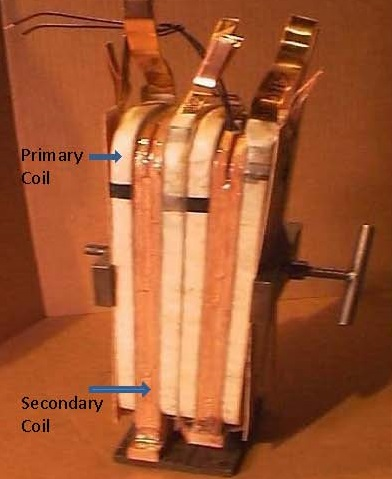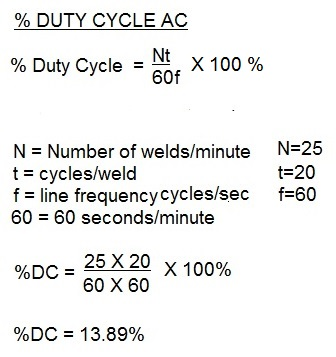# Controls & Transformers

STEP 1
Calculate the duty cycle at 50%.STEP 2
With the duty cycle calculated, use step 2 to calculate the actual power required to operate at the 50% duty cycle. Then compare the values to determine if the 70KVA transformer is sufficient for the job.

KVA demand is a measure of the actual instantaneous demand for power placed on the electrical delivery system of the plant during the weld cycle. The KVA Demand in turn is used to calculate the KVA rating at 50% duty cycle. Transformers are commonly rated at 50% duty cycle.
Below is an example of a calculation of KVA demand for an application using a 70 KVA AC transformer to determine if it will be adequate?

Resistance welding transformers are very robust. They can operate for years without any problems. When they fail it is catastrophic. A short between the primary and secondary coils is one method of failure. This occurs when the insulation between these coils fails. It is a very thin separation between the primary and secondary coils. Any damage to this thin layer can lead to a catastrophic short between the two coils. To prevent this from on the replacement transformer the cause for the short must be sought and a plan to prevent in the future put into place.Transformer Coils Assembled showing primary and secondary coils.                                Simpolified Sketch of The Power Components

The transformer turns ratio in resistance welding reflects the number of secondary coil turns versus the number of primary coil turns. In AC transformers, there are many turns in the primary. The secondary has one turn. If there are 50 turns then the ratio is 50/1. Therefor the primary voltage will drop from 220 V and 100 amp input to 220/50 = 4.4 volts in the secondary. The equations for this are:

Duty cycle is a measurement of the % of the time that the transformer is conducting current during one minute. This value is used to insure that the electrical components are not operating above their thermal capability. Resistance welding transformers are rated at a 50% duty cycle. Each application may operate at different duty cycle up to and including 100% continuous.

THE FORMULA TO CALCULATE DUTY CYCLE FOR AC is:THE FORMULA TO CALCULATE DUTY CYCLE FOR MFDC is:Reference:  RWMA Manual Chapter 19

Roman Manufacturing Inc.

### Have a Question?

Do you have a question that is not covered in our knowledgebase? Do you have questions regarding the above article? Click here to ask the professor.